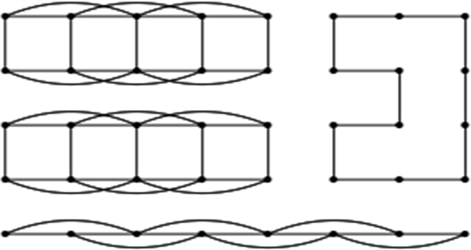# 几类笛卡尔乘积图的k路顶点覆盖数问题The k-Path Vertex Cover in Several Cartesian Product Graphs

• 全文下载: PDF(555KB)    PP.1182-1186   DOI: 10.12677/AAM.2017.69143
• 下载量: 744  浏览量: 2,352

For a graph G and a positive integer k, a subset S of vertices of G is called a k-path vertex cover if S intersects all paths of order k in G. The cardinality of a minimum k-path vertex cover is denoted by φk(G), and is called the k-path vertex cover number of G. In this paper, we study some Cartesian products and give several estimations of φk(CmPN2).

1. 引言

$k$ 路顶点覆盖问题的概念是首次由Novotný (2010)和Brešar et al. (2011)在研究无线电安全网络的连通问题上被引入的   。涂建华在2011年的交通安全控制问题中也提及到 $k$ 路顶点覆盖问题的概念   。无线电传感网络的发展起源于军事应用并简记为WSNs。无论如何，WSNs现在多用于一些民用的设施，如环境的监控，家庭自动化装置和交通控制。无线电安全网络系统可以用图论的语言来表示其拓扑结构  ，我们用顶点代表传感装置，用边来描述每对传感装置间传递的信号。我们关注 $k$ 概括保护方案，它保证每两个无线设备间传输的数据的完整性。我们的方案假设在连通的网络中次序为 $k$ 的传送路中至少有一个装置被保护  。本文我们主要研究几类关于圈和路的笛卡尔乘积图的最小的 $k$ 路顶点覆盖数。

2. 相关的引理

$\begin{array}{l}{\phi }_{k}\left({P}_{n}\right)=⌊\frac{n}{k}⌋,\\ {\phi }_{k}\left({C}_{n}\right)=⌈\frac{n}{k}⌉,\\ {\phi }_{k}\left({K}_{n}\right)=n-k+1.\end{array}$

(2) 假设 $S$$G$ 的一个 $k$ 路顶点覆盖集， ${G}^{\prime }$ 是图 $G$ 的一个子图，那么 $S\cap V\left(G\right)$ 是图 ${G}^{\prime }$ 的一个 $k$ 路顶点覆盖集，由于 $|S\cap V\left({G}^{\prime }\right)|\le |S|$ ，于是 ${\phi }_{k}\left({G}^{\prime }\right)\le {\phi }_{k}\left(G\right)$

3. ${P}_{m}$${C}_{n}$ 的笛卡尔乘积图的最小的k路顶点覆盖数的上界

${\phi }_{k}\left({P}_{m}\square {C}_{n}\right)\le \mathrm{min}\left\{m⌈\frac{n}{a+1}⌉+n⌊\frac{m}{b+1}⌋-2⌈\frac{n}{a+1}⌉⌊\frac{m}{b+1}⌋,m⌈\frac{n}{b+1}⌉+n⌊\frac{m}{a+1}⌋-2⌈\frac{n}{b+1}⌉⌊\frac{m}{a+1}⌋\right\}$

${S}_{1}=\left\{\left({u}_{i},{v}_{j}\right)\in {P}_{m}\square {C}_{n}|i\in \left[1,m\right],j\equiv 0\left(\mathrm{mod}\left(a+1\right)\right)\right\}\cup \left\{\left({u}_{i},{v}_{n}\right)\in {P}_{m}\square {C}_{n}|对于固定系数n\right\}$

${S}_{2}=\left\{\left({u}_{i},{v}_{j}\right)\in {P}_{m}\square {C}_{n}|j\in \left[1,m\right],i\equiv 0\left(\mathrm{mod}\left(b+1\right)\right)\right\}$ .

${}^{{u}_{i}}C{}_{n}$ 中我们覆盖了每一个第 $a+1$ 个顶点和第 $n$ 个顶点，由于有 $m$ 层，所以 $|{S}_{1}|=m⌈\frac{n}{a+1}⌉$ 。同理，我们有 $|{S}_{2}|=n⌊\frac{m}{b+1}⌋$ ，由于我们把每个交叉的位置处的顶点算了两次，而且 $|{S}_{1}\cap {S}_{2}|=⌈\frac{n}{a+1}⌉⌊\frac{m}{b+1}⌋$ ，所以覆盖集 $S$ 的大小为

$|S|=m⌈\frac{n}{a+1}⌉+n⌊\frac{m}{b+1}⌋-2⌈\frac{n}{a+1}⌉⌊\frac{m}{b+1}⌋$ .

4. ${C}_{m}$${P}_{n}^{2}$ 的笛卡尔乘积图的最小的k路顶点覆盖数的下界

${\phi }_{k}\left({C}_{m}\square {P}_{n}^{2}\right)\ge \left\{\begin{array}{l}4⌊\frac{m}{2}⌋⌊\frac{n}{k+1}⌋+⌈\frac{\left(m-1\right)\left(n-⌊\frac{n}{k+1}⌋\right)}{k}⌉+2⌊\frac{n}{k+1}⌋,\text{\hspace{0.17em}}如果m为奇数\\ 4⌊\frac{m}{2}⌋⌊\frac{n}{k+1}⌋+⌈\frac{\left(m-1\right)\left(n-⌊\frac{n}{k+1}⌋\right)}{k}⌉,\text{\hspace{0.17em}}如果m为偶数\end{array}$

${\phi }_{k}\left({C}_{m}\square {P}_{n}^{2}\right)\ge 4⌊\frac{m}{2}⌋⌊\frac{n}{k+1}⌋+⌈\frac{\left(m-1\right)\left(n-⌊\frac{n}{k+1}⌋\right)}{k}⌉+2⌊\frac{n}{k+1}⌋$ .Figure 1. A partition of ${C}_{m}\square {P}_{n}^{2}$ for odd m

${\phi }_{k}\left({C}_{m}\square {P}_{n}^{2}\right)\ge 4⌊\frac{m}{2}⌋⌊\frac{n}{k+1}⌋+⌈\frac{\left(m-1\right)\left(n-⌊\frac{n}{k+1}⌋\right)}{k}⌉$ .

${\phi }_{k}\left({C}_{m}\square {P}_{n}^{2}\right)\ge 4⌊\frac{m}{2}⌋⌊\frac{n}{k+1}⌋+⌈\frac{\left(mn-\left(m-1\right)⌊\frac{n}{k+1}⌋\right)}{k}⌉$ .

$\begin{array}{c}{\phi }_{k}\left({C}_{m}\square {P}_{n}^{2}\right)\ge x{\phi }_{k}\left({P}_{2}\square {P}_{k+1}^{2}\right)+y{\phi }_{k}\left({C}_{y}\right)=4x+⌊\frac{y}{k}⌋\\ =4⌊\frac{m}{2}⌋⌊\frac{n}{k+1}⌋+⌈\frac{\left(mn-\left(m-1\right)⌊\frac{n}{k+1}⌋\right)}{k}⌉.\end{array}$

  Xiao, M.Y. and Kou, S.W. (2017) Exact Algorithms for the Maximum Dissociation Set and Minimum 3-Path Vertex Cover problems. Theoretical Computer Science, 657, 86-97. https://doi.org/10.1016/j.tcs.2016.04.043  Brešar, B., Kardoš, F., Katrenič, J. and Semanišin, G. (2011) Minimum k-Path Vertex Cover. Discrete Applied Mathematics, 159, 1189-1195. https://doi.org/10.1016/j.dam.2011.04.008  Novotný, M. (2010) Design and Analysis of a Generalized Canvas Protocol, Information Security Theory and Practices. Security and Privacy of Pervasive Systems and Smart Devices, 6033, Springer, 106-121.  Tu, J. (2015) A Fixed-Parameter Algorithm for the Vertex Cover Problem. Information Processing Letters, 115, 96-99. https://doi.org/10.1016/j.ipl.2014.06.018  Tu, J.H. and Zhou, W.L. (2011) A Primal-Dual Approximation Algorithm for the Vertex Cover P3 Problem. Theoretical Computer Science, 412, 7044-7048. https://doi.org/10.1016/j.tcs.2011.09.013  Bondy, J.A. and Murty, U.S.R. (1976) Graph Theory with Appli-cation. M. The Macmillan Press Ltd. https://doi.org/10.1007/978-1-349-03521-2  Novotny, M. (2010) Design and Analysis of a Generalized Canvas Protocol. In: Proceedings of WISTP 2010, LNCS, Vol. 6033, Springer-Verlag, 106-121. https://doi.org/10.1007/978-3-642-12368-9_8  Kardoš, F., Katrenič, J. and Schiermeyer, I. (2011) On Com-puting the Minimum 3-Path Vertex Cover and Dissociation Number of Graphs. Theoretical Computer Science, 412, 7009-7017. https://doi.org/10.1016/j.tcs.2011.09.009